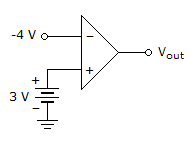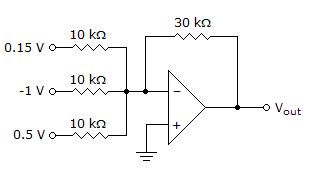# Electronic Devices - Basic Op-Amp Circuits

### Exercise :: Basic Op-Amp Circuits - General Questions

16.

A good example of hysteresis is a(n)

 A. AM radio. B. thermostat. C. alarm clock. D. none of the above

Explanation:

No answer description available for this question. Let us discuss.

17.

To reduce the effects of noise resulting in erratic switching of output states of a comparator, you can use

 A. the upper trigger point. B. the lower trigger point. C. nonzero-level detection. D. hysteresis.

Explanation:

No answer description available for this question. Let us discuss.

18.

Refer to the given figure. With the inputs shown, determine the output voltage.A. 7 V B. –7 V C. +Vsat D. –Vsat

Explanation:

No answer description available for this question. Let us discuss.

19.

Refer to the given figure. Determine the output voltage, VOUT.A. 1.05 V B. –0.35 V C. 0.35 V D. –1.05 V

Explanation:

No answer description available for this question. Let us discuss.

20.

What is (are) the necessary component(s) for the design of a bounded comparator?

 A. rectifier diodes B. zener diodes C. both of the above